# FSC Math Full Book MCQs 1

NUST entry test 2023 NET contains most of the Mathematics MCQs. FSC Part 2 Math book MCQs. HSSC Math questions MCQs. Solved Chapterwise Math MCQs for first-year 12 class MCQs. Second-year and first-year Math textbooks chapter-wise MCQs with the answer are provided here. PakLearningSpot PLS MCQs Bank website for FREE Entry test preparations

0%
683
Created by Ali Durrani

NET Practice

Math Practice Test 1

1 / 100

Category: Math Ch 3 Class 12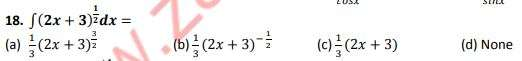2 / 100

Category: Math Ch 9

One right angle is equal to

3 / 100

Category: Math Ch 4

Solution set of the equation 𝒙^𝟐 − 𝟒𝒙 + 𝟒 = 𝟎 is:

4 / 100

Category: Math Ch 105 / 100

Category: Math Ch 9

𝟏𝟔°𝟑𝟎′ equal to

6 / 100

Category: Math Ch 12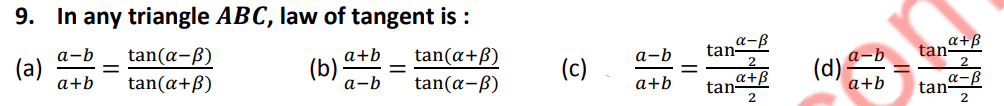7 / 100

Category: Math Ch 7

Non occurrence of an event E is denoted by:

8 / 100

Category: Math Ch 7 Class 12

Parallelogram law of vector addition to describe the combined action of two forces, was used
by

9 / 100

Category: Math Ch 4 Class 12

A four sided polygon (quadrilateral) having two parallel and non-parallel sides is called

10 / 100

Category: Math

Every function is:

11 / 100

Category: Math Ch 13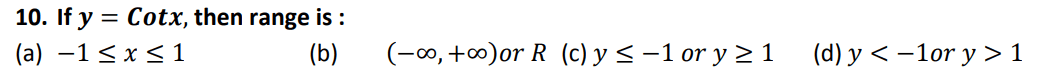12 / 100

Category: Math Ch 7 Class 12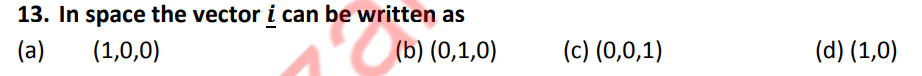13 / 100

Category: Math Ch 7 Class 12

The angle in semi-circle is equal to:

14 / 100

Category: Math Ch 9

If the rotation of the angle is counter clock wise, then angle is:

15 / 100

Category: Math Ch 416 / 100

Category: Math Ch 10

Distance between the points 𝑨(𝟑, 𝟖) & 𝐵(5,6) is:

17 / 100

Category: Math Ch 12

Which can be reduced to Pythagoras theorem,

18 / 100

Category: Math Ch 1419 / 100

Category: Math Ch 14

All trigonometric functions are ……………….. functions.

20 / 100

Category: Math Ch 1421 / 100

Category: Math Ch 3

6. A matrix A is called real if all 𝒂𝒊𝒋 are

22 / 100

Category: Math Ch 12

When we look an object below the horizontal ray, the angle formed is called angle of:
(a) Elevation (b) ✔ depression (c) incidence (d) reflects

23 / 100

Category: Math Ch 4 Class 12

The straight line which passes through one vertex and though the mid-point of the opposite
side is called:

24 / 100

Category: Math Ch 4 Class 12

The slope of the line ?? + ?? + ? = ? is

25 / 100

Category: Math Ch 7 Class 1226 / 100

Category: Math Ch 13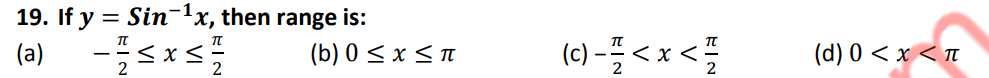27 / 100

Category: Math Ch 3 Class 12

The equation 𝒚 = 𝒙𝟐 − 𝟐𝒙 + 𝒄 represents ( 𝒄 being a parameter )

28 / 100

Category: Math Ch 12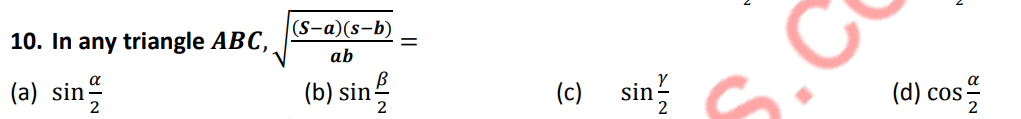29 / 100

Category: Math Ch 2 Class 12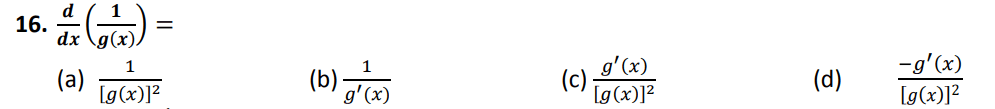30 / 100

Category: Math Ch 4 Class 12

If line ? intersects ? − ???? at a point (?, ?) , then the ? − ????????? of the line ? is:

31 / 100

Category: Math Ch 10

𝒔𝒊𝒏(𝟐𝝅 − 𝜽) =

32 / 100

Category: Math Ch 4 Class 12

The point where the angle bisectors of a triangle intersect is called_________ of the triangle.

33 / 100

Category: Math Ch 7 Class 12

The vector whose magnitude is 1 is called

34 / 100

Category: Math Ch 6

The last term of an infinite sequence is called :

35 / 100

Category: Math Ch 1

(−𝟏)^−𝟐𝟏/𝟐 =

36 / 100

Category: Math Ch 7 Class 1237 / 100

Category: Math Ch 6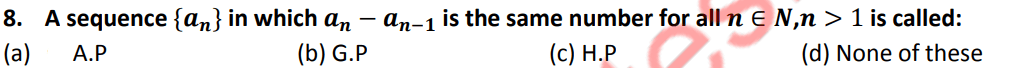38 / 100

Category: Math Ch 2 Class 12

The maximum value of the function ?(?) = ?^? − ? − ? is

39 / 100

Category: Math Ch 3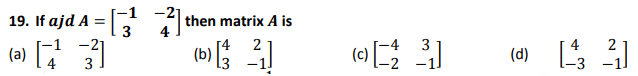40 / 100

Category: Math Ch 3 Class 1241 / 100

Category: Math Ch 1342 / 100

Category: Math Ch 643 / 100

Category: Math Ch 3

53. The inverse of unit matrix is:

44 / 100

Category: Math Ch 7 Class 12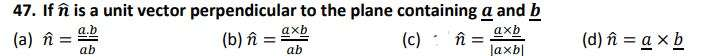45 / 100

Category: Math Ch 5 Class 12

Solution space consisting of all feasible solutions of system of linear in inequalities is called

46 / 100

Category: Math Ch 3 Class 12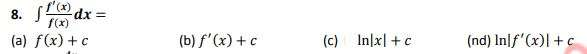47 / 100

Category: Math Ch 5 Class 1248 / 100

Category: Math Ch 1 Class 12

When we say that 𝒇 is function from set 𝑿 to set 𝒀, then 𝑿 is called

49 / 100

Category: Math Ch 6 Class 12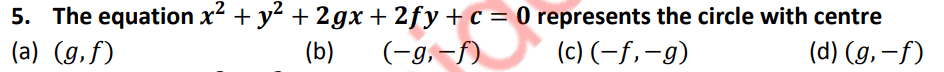50 / 100

Category: Math Ch 3

The element 𝒂𝒊𝒋 of any matrix A is present in:

51 / 100

Category: Math Ch 7 Class 12

The angles 𝜶, 𝜷, 𝒂𝒏𝒅 𝜸 which a non-zero vector 𝒓 makes with 𝒙 − 𝒂𝒙𝒊𝒔, 𝒚 − 𝒂𝒙𝒊𝒔 and
𝒛 − 𝒂𝒙𝒊𝒔 respectively are called_____________ of 𝒓.

52 / 100

Category: Math Ch 3

The main diagonal elements of a skew hermitian matrix must be:

53 / 100

Category: Math Ch 7 Class 1254 / 100

Category: Math Ch 9

3600th part of 𝟏° is equal to

55 / 100

Category: Math Ch 7 Class 12

The vector whose initial point is at the origin and terminal point is ? , is called

56 / 100

Category: Math

Inverse of a line is :

57 / 100

Category: Math Ch 6

The general term of a ?. ?., is :

58 / 100

Category: Math Ch 4 Class 12

The Normal form of the equation of the straight line is

59 / 100

Category: Math Ch 6 Class 12

The locus of a revolving line with one end fixed and other end on the circumference of a circle
of a circle is called:

60 / 100

Category: Math Ch 6 Class 12

. Circle is the special case of :

61 / 100

Category: Math Ch 3 Class 12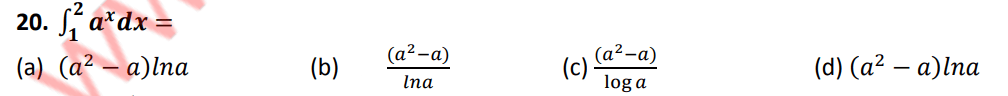62 / 100

Category: Math Ch 2

Identity element in (𝑪, . ) is:

63 / 100

Category: Math Ch 12

A “Triangle” has :

64 / 100

Category: Math Ch 2

If 𝒙 ∈ 𝑩′ = 𝑼 − 𝑩 then

65 / 100

Category: Math Ch 7 Class 12

If any two vectors of scalar triple product are equal, then its value is equal to

66 / 100

Category: Math Ch 767 / 100

Category: Math Ch 1

𝒊^𝟏𝟑 equals:

68 / 100

Category: Math Ch 5 Class 12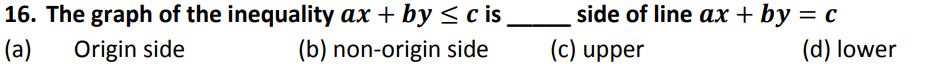69 / 100

Category: Math

√3 is __________

70 / 100

Category: Math Ch 7

. The number of ways for sitting 4 persons in a train on a straight sofa is:

71 / 100

Category: Math

The multiplicative identity of complex number is

72 / 100

Category: Math Ch 3 Class 12

The equation 𝒚 = 𝒙𝟐 − 𝟐𝒙 + 𝒄 represents ( 𝒄 being a parameter )
(Error Comment below the anwser)

73 / 100

Category: Math Ch 3 Class 12

The order of the differential equation : ????/??? +??/?? − ? = ?

74 / 100

Category: Math Ch 13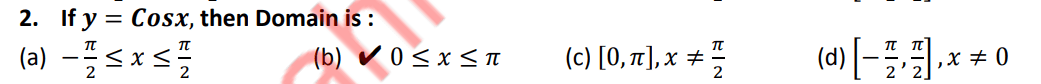75 / 100

Category: Math Ch 4 Class 12

If the equations of the sides of a triangle are given then the intersection of any two lines in
pairs gives ________ the triangles.

76 / 100

Category: Math Ch 777 / 100

Category: Math Ch 7 Class 12

Two vectors are said to be negative of each other if they have the same magnitude and
__________direction.

78 / 100

Category: Math

A set is a collection of objects which are

79 / 100

Category: Math Ch 7 Class 1280 / 100

Category: Math Ch 11

Range of 𝒚 = 𝒕𝒂𝒏𝒙 is

81 / 100

Category: Math Ch 682 / 100

Category: Math Ch 3

9. If a matrix A has same number of rows and columns then A is called:

83 / 100

Category: Math Ch 3

If a matrix A has same number of rows and columns then A is called:

84 / 100

Category: Math Ch 4 Class 12

The lines lying in the same plane are called

85 / 100

Category: Math Ch 786 / 100

Category: Math Ch 3

The matrix  is :

87 / 100

Category: Math

##### A decimal which has a finite numbers of digits in its decimal part is calleddecimal.

(b)     (c)                  (d)

88 / 100

Category: Math Ch 6

If sum of series is not defined then it is called:

89 / 100

Category: Math Ch 4

The sum of all four fourth roots of unity is:

90 / 100

Category: Math Ch 7 Class 12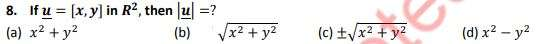91 / 100

Category: Math Ch 592 / 100

Category: Math Ch 893 / 100

Category: Math Ch 5

When a rational fraction is separated into partial fractions, then result is always :

94 / 100

Category: Math Ch 2 Class 1295 / 100

Category: Math Ch 2 Class 12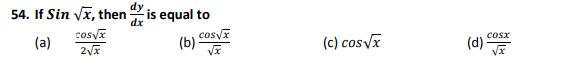96 / 100

Category: Math Ch 14

An equation containing at least one trigonometric function is called:

97 / 100

Category: Math Ch 1

Golden rule of fraction is that for 𝒌 ≠ 𝟎, 𝒂/𝒃 =

98 / 100

Category: Math Ch 11

Range of 𝒚 = 𝒔𝒊𝒏𝒙 is

99 / 100

Category: Math Ch 7 Class 12

Which of the following can be the direction angles of some vector

100 / 100

Category: Math Ch 14

12. General solution of every trigonometric equation consists of :

The average score is 40%

0%

### 9 responses to “FSC Math Full Book MCQs 1”

1. Shahzaib khan says:

Why the questions are incomplete

2. Sabeeh says:

The numbers are not visible
Its all not useful. Change it

3. Saboor is says:

The numbers are not showing in each MCQS it is showing ? instead of numbers

4. Anonymous says:

The question is incomplete

5. Anonymous says:

Ghbhhh

6. Anonymous says:

The numbers seem to be missing. It shows a question mark symbol instead of numbers.

7. Anonymous says:

Fix ittttttttt

Salam